# Cnoidal wave

Cnoidal waveUS Army bombers flying over near-periodic swell in shallow water, close to the Panama coast (1933). The sharp crests and very flat troughs are characteristic for cnoidal waves.

In fluid dynamics, a cnoidal wave is a nonlinear and exact periodic wave solution of the Korteweg–de Vries equation. These solutions are in terms of the Jacobi elliptic function cn, which is why they are coined cnoidal waves. They are used to describe surface gravity waves of fairly long wavelength, as compared to the water depth.

The cnoidal wave solutions were derived by Korteweg and de Vries, in their 1895 paper in which they also propose their dispersive long-wave equation, now known as the Korteweg–de Vries equation. In the limit of infinite wavelength, the cnoidal wave becomes a solitary wave.

The Benjamin–Bona–Mahony equation has improved short-wavelength behaviour, as compared to the Korteweg–de Vries equation, and is another uni-directional wave equation with cnoidal wave solutions. Further, since the Korteweg–de Vries equation is an approximation to the Boussinesq equations for the case of one-way wave propagation, cnoidal waves are approximate solutions to the Boussinesq equations.

Cnoidal wave solutions can appear in other applications than surface gravity waves as well, for instance to describe ion acoustic waves in plasma physics.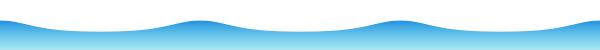A cnoidal wave, characterised by sharper crests and flatter troughs than in a sine wave. For the shown case, the elliptic parameter is m = 0.9.

## Background

### Korteweg–de Vries, and Benjamin–Bona–Mahony equations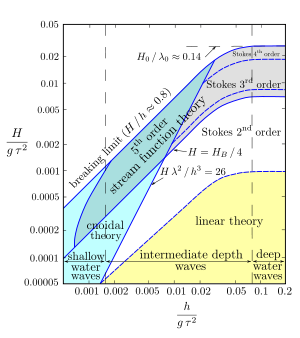Validity of several theories for periodic water waves, according to Le Méhauté (1976). The light-blue area gives the range of validity of cnoidal wave theory; light-yellow for Airy wave theory; and the dashed blue lines demarcate between the required order in Stokes' wave theory. The light-gray shading gives the range extension by numerical approximations using fifth-order stream-function theory, for high waves (H > ¼ Hbreaking).

The Korteweg–de Vries equation (KdV equation) can be used to describe the uni-directional propagation of weakly nonlinear and long waves—where long wave means: having long wavelengths as compared with the mean water depth—of surface gravity waves on a fluid layer. The KdV equation is a dispersive wave equation, including both frequency dispersion and amplitude dispersion effects. In its classical use, the KdV equation is applicable for wavelengths λ in excess of about five times the average water depth h, so for λ > 5 h; and for the period τ greater than$7 \sqrt{h/g}$ with g the strength of the gravitational acceleration. To envisage the position of the KdV equation within the scope of classical wave approximations, it distinguishes itself in the following ways:

• Korteweg–de Vries equation — describes the forward propagation of weakly nonlinear and dispersive waves, for long waves with λ > 7 h.
• Shallow water equations — are also nonlinear and do have amplitude dispersion, but no frequency dispersion; they are valid for very long waves, λ > 20 h.
• Boussinesq equations — have the same range of validity as the KdV equation (in their classical form), but allow for wave propagation in arbitrary directions, so not only forward-propagating waves. The drawback is that the Boussinesq equations are often more difficult to solve than the KdV equation; and in many applications wave reflections are small and may be neglected.
• Airy wave theory — has full frequency dispersion, so valid for arbitrary depth and wavelength, but is a linear theory without amplitude dispersion, limited to low-amplitude waves.
• Stokes' wave theory — a perturbation-series approach to the description of weakly nonlinear and dispersive waves, especially successful in deeper water for relative short wavelengths, as compared to the water depth. However, for long waves the Boussinesq approach—as also applied in the KdV equation—is often preferred. This is because in shallow water the Stokes' perturbation series needs many terms before convergence towards the solution, due to the peaked crests and long flat troughs of the nonlinear waves. While the KdV or Boussinesq models give good approximations for these long nonlinear waves.

The KdV equation can be derived from the Boussinesq equations, but additional assumptions are needed to be able to split-off of the forward wave propagation. For practical applications, the Benjamin–Bona–Mahony equation (BBM equation) is preferable over the KdV equation, a forward-propagating model similar to KdV but with much better frequency-dispersion behaviour at shorter wavelengths. Further improvements in short-wave performance can be obtained by starting to derive a one-way wave equation from a modern improved Boussinesq model, valid for even shorter wavelengths.

### Cnoidal waves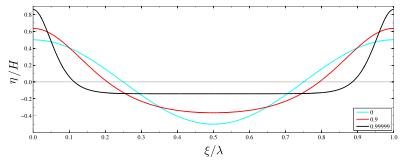Cnoidal wave profiles for three values of the elliptic parameter m.
 blue : m = 0, red : m = 0.9 and black : m = 0.99999.

The cnoidal wave solutions of the KdV equation were presented by Korteweg and de Vries in their 1895 paper, which article is based on the PhD thesis by de Vries in 1894. Solitary wave solutions for nonlinear and dispersive long waves had been found earlier by Boussinesq in 1872, and Rayleigh in 1876. The search for these solutions was triggered by the observations of this solitary wave (or "wave of translation") by Russell, both in nature and laboratory experiments. Cnoidal wave solutions of the KdV equation are stable with respect to small perturbations.

The surface elevation η(x,t), as a function of horizontal position x and time t, for a cnoidal wave is given by:$\eta(x,t) = \eta_2 + H\, \operatorname{cn}^2\, \left( \begin{array}{c|c} \displaystyle 2\, K(m)\, \frac{x-c\,t}{\lambda} & m \end{array} \right),$

where H is the wave height, λ is the wavelength, c is the phase speed and η2 is the trough elevation. Further cn is one of the Jacobi elliptic functions and K(m) is the complete elliptic integral of the first kind; both are dependent on the elliptic parameter m. The latter, m, determines the shape of the cnoidal wave. For m equal to zero the cnoidal wave becomes a cosine function, while for values close to one the cnoidal wave gets peaked crests and (very) flat troughs.

An important dimensionless parameter for nonlinear long waves (λ  h) is the Ursell parameter:$U = \frac{H\, \lambda^2}{h^3} \qquad = \frac{H}{h}\, \left( \frac{\lambda}{h} \right)^2.$

For small values of U, say U < 5, a linear theory can be used, and at higher values nonlinear theories have to be used, like cnoidal wave theory. The demarcation zone between—third or fifth order—Stokes' and cnoidal wave theories is in the range 10–25 of the Ursell parameter. As can be seen from the formula for the Ursell parameter, for a given relative wave height H/h the Ursell parameter—and thus also the nonlinearity—grows quickly with increasing relative wavelength λ/h.

Based on the analysis of the full nonlinear problem of surface gravity waves within potential flow theory, the above cnoidal waves can be considered the lowest-order term in a perturbation series. Higher-order cnoidal wave theories remain valid for shorter and more nonlinear waves. A fifth-order cnoidal wave theory was developed by Fenton in 1979. A detailed description and comparison of fifth-order Stokes' and fifth-order cnoidal wave theories is given in the review article by Fenton.

Cnoidal wave descriptions, through a renormalisation, are also well suited to waves on deep water, even infinite water depth; as found by Clamond. A description of the interactions of cnoidal waves in shallow water, as found in real seas, has been provided by Osborne in 1994.

## Periodic wave solutions

### Korteweg–de Vries equation

The Korteweg–de Vries equation (KdV equation), as used for water waves and in dimensional form, is:$\partial_t \eta + \sqrt{gh}\; \partial_x \eta + \tfrac{3}{2}\, \sqrt{\frac{g}{h}}\; \eta\, \partial_x \eta + \tfrac{1}{6}\, h^2\, \sqrt{gh}\; \partial_x^3 \eta = 0,$

where

 η : surface elevation, a function of x and t, with the positive direction upwards (opposing gravity), x : horizontal coordinate, t : time, g : the value of Earth's gravity, h : the mean water depth, and ∂x and ∂t : partial derivative operators with respect to x and t.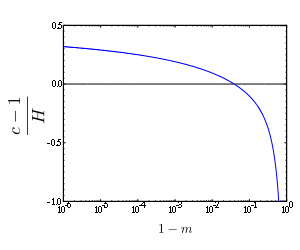Relative phase speed increase of cnoidal wave solutions for the Korteweg–de Vries equation as a function of 1−m, with m the elliptic parameter.
The horizontal axis is on a logarithmic scale, from 10−6 to 100=1.
The figure is for non-dimensional quantities, i.e. the phase speed c is made dimensionless with the shallow-water phase speed$\sqrt{gh}$, and the wave height H is made dimensionless with the mean water depth h.

The cnoidal-wave solution of the KdV equation is:$\eta(x,t) = \eta_2 + H\, \operatorname{cn}^2 \left( \begin{array}{c|c} \displaystyle \frac{x-c\,t}{\Delta} & m \end{array} \right),$

with H the wave height—the difference between crest and trough elevation, η2 the trough elevation, m the elliptic parameter, c the phase speed and cn one of the Jacobi elliptic functions. The trough level η2 and width parameter Δ can be expressed in terms of H, h and m:$\eta_2 = \frac{H}{m}\, \left( 1 - m - \frac{E(m)}{K(m)} \right),$   and$\Delta=\frac{\lambda}{2\,K(m)}\, = h\, \sqrt{\frac{4}{3}\frac{m\, h}{H}},$

with K(m) the complete elliptic integral of the first kind and E(m) the complete elliptic integral of the second kind. Note that K(m) and E(m) are denoted here as a function of the elliptic parameter m and not as a function of the elliptic modulus k, with m = k2.

The wavelength λ, phase speed c and wave period τ are related to H, h and m by:$\lambda = h\, \sqrt{\frac{16}{3}\frac{m\,h}{H}}\; K(m),$$c = \sqrt{gh}\, \left[ 1 + \frac{H}{m\, h}\, \left( 1 - \frac12\, m - \frac32\, \frac{E(m)}{K(m)} \right) \right]$   and$\tau = \frac{\lambda}{c},$

with g the Earth's gravity.

Most often, the known wave parameters are the wave height H, mean water depth h, gravitational acceleration g, and either the wavelength λ or else the period τ. Then the above relations for λ, c and τ are used to find the elliptic parameter m. This requires numerical solution by some iterative method.

### Benjamin–Bona–Mahony equation

The Benjamin–Bona–Mahony equation (BBM equation), or regularised long wave (RLW) equation, is in dimensional form given by:$\partial_t \eta + \sqrt{g\,h}\, \partial_x \eta + \tfrac32\, \sqrt{\frac{g}{h}}\, \eta\, \partial_x \eta - \tfrac16\, h^2\, \partial_t\, \partial_x^2 \eta = 0.$

All quantities have the same meaning as for the KdV equation. The BBM equation is often preferred over the KdV equation because it has a better short-wave behaviour.

The cnoidal wave solution of the BBM equation, together with the associated relationships for the parameters is:\begin{align} \eta(x,t) &= \eta_2 + H\, \operatorname{cn}^2 \left( \begin{array}{c|c} \displaystyle \frac{x-c\,t}{\Delta} & m \end{array} \right), \\ \eta_2 &= \frac{H}{m}\, \left( 1 - m - \frac{E(m)}{K(m)} \right), \\ \Delta &= h\, \sqrt{\frac{4}{3}\, \frac{m\, h}{H}\, \frac{c}{\sqrt{g\, h}} } && = \frac{\lambda}{2\, K(m)}, \\ \lambda &= h\, \sqrt{\frac{16}{3}\, \frac{m\, h}{H}\, \frac{c}{\sqrt{gh}}}\; K(m), \\ c &= \sqrt{gh}\, \left[ 1 + \frac{H}{m\, h}\, \left( 1 - \frac12\, m - \frac32\, \frac{E(m)}{K(m)} \right) \right] && \text{and} \\ \tau &= \frac{\lambda}{c}. \end{align}

The only difference with the cnoidal wave solution of the KdV equation is in the equation for the wavelength λ. For practical applications, usually the water depth h, wave height H, gravitational acceleration g, and either the wavelength λ, or—most often—the period (physics) τ are provided. Then the elliptic parameter m has to be determined from the above relations for λ, c and τ through some iterative method.

## Example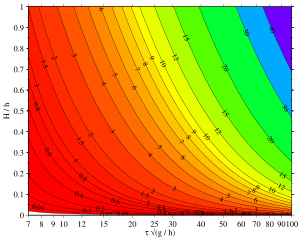Parameter relations for cnoidal wave solutions of the Korteweg–de Vries equation. Shown is −log10 (1−m), with m the elliptic parameter of the complete elliptic integrals, as a function of dimensionless period τ √(g/h) and relative wave height H / h. The values along the contour lines are −log10 (1−m), so a value 1 corresponds with m = 1 − 10−1 = 0.9 and a value 40 with m = 1 − 10−40.

In this example, a cnoidal wave according to the Korteweg–de Vries (KdV) equation is considered. The following parameters of the wave are given:

Instead of the period τ, in other cases the wavelength λ may occur as a quantity known beforehand.

First, the dimensionless period is computed:$\tau\, \sqrt{\frac{g}{h}} = 9.80,$

which is larger than seven, so long enough for cnoidal theory to be valid. The main unknown is the elliptic parameter m. This has to be determined in such a way that the wave period τ, as computed from cnoidal wave theory for the KdV equation:$\lambda = h\, \sqrt{\frac{16}{3}\frac{m\,h}{H}}\; K(m),$$c = \sqrt{gh}\, \left[ 1 + \frac{H}{m\, h}\, \left( 1 - \frac12\, m - \frac32\, \frac{E(m)}{K(m)} \right) \right]$   and$\tau = \frac{\lambda}{c},$

is consistent with the given value of τ; here λ is the wavelength and c is the phase speed of the wave. Further, K(m) and E(m) are complete elliptic integrals of the first and second kind, respectively. Searching for the elliptic parameter m can be done by trial and error, or by use of a numerical root-finding algorithm. In this case, starting from an initial guess minit = 0.99, by trial and error the answer$m = 0.9832\,$

is found. Within the process, the wavelength λ and phase speed c have been computed:

• wavelength λ = 50.8 m (167 ft), and
• phase speed c = 7.26 m/s (23.8 ft/s).

The phase speed c can be compared with its value$\sqrt{gh}$ according to the shallow water equations:$\frac{c}{\sqrt{g\, h}} = 1.0376,$

showing a 3.8% increase due to the effect of nonlinear amplitude dispersion, which wins in this case from the reduction of phase speed by frequency dispersion.

Now the wavelength is known, the Ursell number can be computed as well:$U = \frac{H\, \lambda^2}{h^3} = 62,$

which is not small, so linear wave theory is not applicable, but cnoidal wave theory is. Finally, the ratio of wavelength to depth is λ / h = 10.2 > 7, again indicating this wave is long enough to being considered as a cnoidal wave.

## Solitary-wave limit

For very long nonlinear waves, with the parameter m close to one, m → 1, the Jacobi elliptic function cn can be approximated by$\operatorname{cn} \left( z | m \right) \approx \operatorname{sech}(z) - \tfrac14\, (1-m)\, \Bigl[ \sinh(z)\; \cosh(z) - z \Bigr]\, \tanh(z)\; \operatorname{sech}(z),$   with$\operatorname{sech}(z) = \frac{1}{\cosh(z)}.$

Here sinh, cosh, tanh and sech are hyperbolic functions. In the limit m = 1:$\operatorname{cn} \left( z | m \right) \to \operatorname{sech}(z),$

with sech(z) = 1 / cosh(z).

Further, for the same limit of m → 1, the complete elliptic integral of the first kind K(m) goes to infinity, while the complete elliptic integral of the second kind E(m) goes to one. This implies that the limiting values of the phase speed c and minimum elevelation η2 become:$c = \sqrt{g\,h}\, \left( 1 + \frac12\, \frac{H}{h} \right)$   and$\eta_2 = 0.\,$

Consequently, in terms of the width parameter Δ, the solitary wave solution to both the KdV and BBM equation is:$\eta(x,t) = H\, \operatorname{sech}^2 \left( \frac{x - c\, t}{\Delta} \right).$

The width parameter, as found for the cnoidal waves and now in the limit m → 1, is different for the KdV and the BBM equation:$\Delta = h\, \sqrt{\frac{4\,h}{3\,H}}$ : KdV equation, and$\Delta = h\, \sqrt{\frac{4\,h}{3\,H}\, \frac{c}{\sqrt{g\,h}}}$ : BBM equation.

But the phase speed of the solitary wave in both equations is the same, for a certain combination of height H and depth h.

## Limit of infinitesimal wave height

For infinitesimal wave height the results of cnoidal wave theory are expected to converge towards those of Airy wave theory for the limit of long waves λ  h. First the surface elevation, and thereafter the phase speed, of the cnoidal waves for infinitesimal wave height will be examined.

### Surface elevation

For infinitesimal wave height, in the limit m → 0, the free-surface elevation becomes:$\eta(x,t) = \tfrac12\, H\, \cos\, \theta,$   with$\theta = 2\, \pi\, \frac{\xi}{\lambda} = 2\, \pi\ \frac{x-c\,t}{\lambda}.$

So the wave amplitude is ½H, half the wave height. This is of the same form as studied in Airy wave theory, but note that cnoidal wave theory is only valid for long waves with their wavelength much longer than the average water depth.

### Phase speed

The phase speeds for infinitesimal wave height, according to the cnoidal wave theories for the KdV equation and BBM equation, are

 KdV :$c = \Bigl[ 1 - \tfrac16\, \left( \kappa h \right)^2 \Bigr]\, \sqrt{g\,h},$ BBM :$c = \frac{1}{1 + \tfrac16\, \left( \kappa h \right)^2}\, \sqrt{g\,h},$

with κ = 2π / λ the wavenumber and κh the relative wavenumber. These phase speeds are in full agreement with the result obtained by directly searching for sine-wave solutions of the linearised KdV and BBM equations. As is evident from these equations, the linearised BBM equation has a positive phase speed for all κh. On the other hand, the phase speed of the linearised KdV equation changes sign for short waves with κh >$\sqrt{6}$. This is in conflict with the derivation of the KdV equation as a one-way wave equation.

## Direct derivation from the full inviscid-flow equations

Cnoidal waves can be derived directly from the inviscid, irrotational and incompressible flow equations, and expressed in terms of three invariants of the flow, as shown by Benjamin & Lighthill (1954). In a frame of reference moving with the phase speed, in which reference frame the flow becomes a steady flow, the cnoidal wave solutions can directly be related to the mass flux, momentum flux and energy head of the flow. Following Benjamin & Lighthill (1954)—using a stream function description of this incompressible flow—the horizontal and vertical components of the flow velocity are +zΨ and −ξΨ, in the ξ and z direction respectively (ξ = xct). The vertical coordinate z is positive in the upward direction, opposite to the direction of the gravitational acceleration, and the zero level of z is at the impermeable lower boundary of the fluid domain. While the free surface is at z = ζ(ξ); note that ζ is the local water depth, related to the surface elevation η(ξ) as ζ = h + η with h the mean water depth.

In this steady flow, the discharge Q through each vertical cross section is a constant independent of ξ, and because of the horizontal bed also the horizontal momentum flux S, divided by the density ρ, through each vertical cross section is conserved. Further, for this inviscid and irrotational flow, Bernoulli's principle can be applied and has the same Bernoulli constant R everywhere in the flow domain. They are defined as:\begin{align} Q &= \int_0^{\zeta(\xi)} \partial_z \Psi\; \text{d}z, \\ R &= \frac{p}{\rho} + \tfrac12\, \Bigl[ \left( \partial_\xi \Psi \right)^2 + \left( \partial_z \Psi \right)^2 \Bigr] + g\, z \qquad \text{and} \\ S &= \int_0^{\zeta(\xi)} \left[ \frac{p}{\rho} + \left( \partial_z \Psi \right)^2 \right]\; \text{d}z. \end{align}

For fairly long waves, assuming the water depth ζ is small compared to the wavelength λ, the following relation is obtained between the water depth ζ(ξ) and the three invariants Q, R and S:$\tfrac13\, Q^2\, \left( \zeta' \right)^2 \approx -g\, \zeta^3 + 2\, R\, \zeta^2 - 2\, S\, \zeta + Q^2.$

( E )

This nonlinear and first-order ordinary differential equation has cnoidal wave solutions.

For very long waves of infinitesimal amplitude on a fluid of depth h and with a uniform flow velocity v, the flow constants are according to the shallow water equations:$Q_0 = v\, h,$$R_0 = \tfrac{1}{2}\, v^2 + g\,h$   and$S_0 = v^2\, h + \tfrac{1}{2}\, g\, h^2.$

Equation (E) can be brought into non-dimensional form by use of the discharge Q and gravitational acceleration g, and defining the critical depth hc:$h_c = \sqrt{\frac{Q^2}{g}},$

related to the critical flow demarcation between subcritical flow and supercritical flow (see also Froude number). Consequently, the non-dimensional form of the equation is$\tfrac13\, \left( \tilde{\zeta}' \right)^2 \approx -\zeta^3 + 2\, \tilde{R}\, \tilde{\zeta}^2 - 2\, \tilde{S}\, \tilde{\zeta} + 1,$

with$\tilde{\zeta} = \frac{\zeta}{h_c},$$\tilde{\xi} = \frac{\xi}{h_c},$$\tilde{R} = \frac{R}{g\, h_c},$   and$\tilde{S} = \frac{S}{g\, h_c^2}.$

### Derivation

First eliminate the pressure p from the momentum flux S by use of the Bernoulli equation:$S = R\, \zeta - \tfrac12\, g\, \zeta^2 + \int_0^\zeta \tfrac12 \left[ \left( \partial_z \Psi \right)^2 - \left( \partial_\xi \Psi \right)^2 \right]\; \text{d}z.$

The streamfunction Ψ is expanded as a Maclaurin series around the bed at z = 0, and using that the impermeable bed is a streamline and the irrotationality of the flow: Ψ = 0 and ∂z2Ψ = 0 at z = 0:$\Psi = z\, u_b(\xi) - \frac{z^3}{3!}\, u_b''(\xi) + \frac{z^5}{5!}\, u_b^\text{iv}(\xi) + \cdots,$

with ub the horizontal velocity at the bed z = 0. Because the waves are long, h ≫ λ, only terms up to z3 and ζ3 are retained in the approximations to Q and S. The momentum flux S then becomes:$S = R\, \zeta - \tfrac12\, g\, \zeta^2 + \tfrac12\, \zeta\, u_b^2 - \tfrac16\, \zeta^3\, u_b\, u_b'' - \tfrac16\, \zeta^3\, \left( u_b' \right)^2 + \cdots.$

The discharge Q becomes, since it is the value of the streamfunction Ψ at the free surface z = ζ:$Q = \zeta\, u_b(\xi) - \tfrac16\, \zeta^3\, u_b''{\xi} + \cdots.$

As can be seen, the discharge Q is an O(ζ) quantity. From this, the bed velocity is seen to be$u_b = \frac{Q}{\zeta} + \tfrac16\, \zeta^2\, u_b'' + \cdots.$

Note that Q / ζ is an order one quantity. This relation will be used to replace the bed velocity ub by Q and ζ in the momentum flux S. The following terms can be derived from it:\begin{align} u_b^2 &= \frac{Q^2}{\zeta^2} + \tfrac13\, \zeta\, Q\, u_b'' + \cdots, \\ u_b' &= - \frac{Q}{\zeta}\, \zeta' + \tfrac13\, \zeta\, \zeta'\, u_b'' + \tfrac16\, \zeta^2\, u_b''' + \cdots \qquad \text{and} \\ \left( u_b' \right)^2 &= \frac{Q^2}{\zeta^4}\, \left( \zeta' \right)^2 - \tfrac23\, \frac{Q}{\zeta}\, \zeta'\, u_b'' + \cdots. \end{align}

Consequently, the momentum flux S becomes, again retaining only terms up to proportional to ζ3:$S \approx R\, \zeta - \tfrac12\, g\, \zeta^2 + \tfrac12\, \frac{Q^2}{\zeta} - \tfrac16\, \frac{Q^2}{\zeta}\, \left( \zeta' \right)^2.$

Which can directly be recast in the form of equation (E).

## Potential energy

The potential energy density$E_\text{pot} = \frac{1}{\lambda}\, \int_0^\lambda \tfrac12\, \rho\, g\, \eta^2(x,t)\; \text{d}x$

with ρ the fluid density, is one of the infinite number of invariants of the KdV equation. This can be seen by multiplying the KdV equation with the surface elevation η(x,t); after repeated use of the chain rule the result is:$\partial_t \left( \tfrac12\, \eta^2 \right) + \partial_x \left\{ \tfrac12\, \sqrt{g\, h}\, \eta^2 + \tfrac12\, \sqrt{\frac{g}{h}}\, \eta^3 + \tfrac1{12}\, h^2 \sqrt{g\, h}\, \left[ \partial_x^2\left(\eta^2\right) - 3 \left( \partial_x \eta \right)^2 \right] \right\} = 0,$

which is in conservation form, and is an invariant after integration over the interval of periodicity—the wavelength for a cnoidal wave. The potential energy is not an invariant of the BBM equation, but ½ρg [η2 + 16 h2 (x η)2] is.

First the variance of the surface elevation in a cnoidal wave is computed. Note that η2 = −(1/λ0λ H cn2(ξ/Δ|m) dx, cn(ξ/Δ|m)  = cos ψ(ξ) and λ = 2 Δ K(m), so\begin{align} \frac{1}{\lambda}\, \int_0^\lambda \eta^2\; \text{d}x &= \frac{1}{\lambda} \int_0^\lambda \left\{ \eta_2 + H\, \operatorname{cn}^2 \left( \begin{array}{c|c} \displaystyle \frac{\xi}{\Delta} & m\end{array} \right) \right\}^2\; \text{d}\xi = \frac{H^2}{\lambda} \int_0^\lambda \operatorname{cn}^4 \left( \begin{array}{c|c} \displaystyle \frac{\xi}{\Delta} & m\end{array} \right)\; \text{d}\xi - \eta_2^2 \\ &= \frac{\Delta\, H^2}{\lambda} \int_0^{\pi} \cos^4\, \psi\, \frac{\text{d}\xi}{\text{d}\psi}\; \text{d}\psi - \eta_2^2 = \frac{H^2}{2\, K(m)} \int_0^{\pi} \frac{\cos^4\, \psi}{\sqrt{1 - m\, \sin^2\, \psi}}\; \text{d}\psi - \eta_2^2 \\ &= \frac13\, \frac{H^2}{m^2}\, \left[ \left( 2 - 5\, m + 3\, m^2 \right) + \left( 4\, m - 2 \right)\, \frac{E(m)}{K(m)} \right] - \frac{H^2}{m^2}\, \left( 1 - m - \frac{E(m)}{K(m)} \right)^2 \end{align}

The potential energy, both for the KdV and the BBM equation, is subsequently found to be$E_\text{pot} = \tfrac12\, \rho\, g\, H^2\, \left[ - \frac{1}{3\, m} + \frac{2}{3\,m}\, \left( 1 + \frac{1}{m} \right) \left( 1 - \frac{E(m)}{K(m)} \right) - \frac{1}{m^2}\, \left( 1 - \frac{E(m)}{K(m)} \right)^2 \right].$

The infinitesimal wave-height limit (m → 0) of the potential energy is Epot = 116 ρ g H2, which is in agreement with Airy wave theory. The wave height is twice the amplitude, H = 2a, in the infinitesimal wave limit.

## Notes and references

Wikimedia Foundation. 2010.

### Look at other dictionaries:

• cnoidal — adjective Describes a travelling wave whose amplitude is constricted; e.g. a wave in shallow water …   Wiktionary

• Rogue wave — This article is about the deep ocean rogue waves which occur far out at sea. For tsunami and tidal wave phenomena, see those respective articles. For other uses, see Rogue wave (disambiguation). The Draupner wave, a single giant wave measured on… …   Wikipedia

• Wind wave — Ocean wave redirects here. For the film, see Ocean Waves (film). North Pacific storm waves as seen from the NOAA M/V Noble Star, Winter 1989 …   Wikipedia

• Wind wave model — NOAA Wavewatch III 120 hour Forecast for the North Atlantic In fluid dynamics, wind wave modeling describes the effort to depict the sea state and predict the evolution of the energy of wind waves using numerical techniques. These simulations… …   Wikipedia

• Tsunami — For other uses, see Tsunami (disambiguation) …   Wikipedia

• Megatsunami — An artist s rendering of wave shoaling of a tsunami. Megatsunami (also known as iminami (斎波?, purification wave )) …   Wikipedia

• Dispersion (water waves) — This article is about dispersion of waves on a water surface. For other forms of dispersion, see Dispersion (disambiguation). In fluid dynamics, dispersion of water waves generally refers to frequency dispersion, which means that waves of… …   Wikipedia

• Clapotis — Incoming wave (red) reflected at the wall produces the outgoing wave (blue), both being overlaid resulting in the clapotis (black). In hydrodynamics, the clapotis (from French: lapping of water ) is a non breaking standing wave pattern, caused… …   Wikipedia

• Mild-slope equation — Simulation of wave penetration involving diffraction and refraction into Tedious Creek, Maryland, using CGWAVE (which solves the mild slope equation). In fluid dynamics, the mild slope equation describes the combined effects of diffraction and… …   Wikipedia

• Rip current — Riptide redirects here. For other uses, see Riptide (disambiguation). Rip current, as seen from above. The beach is visible at the right top …   Wikipedia

### Share the article and excerpts

##### Direct link
Do a right-click on the link above
and select “Copy Link”